CBSE Class 12 Sample Paper for 2023 Boards

Class 12
Solutions of Sample Papers and Past Year Papers - for Class 12 Boards

##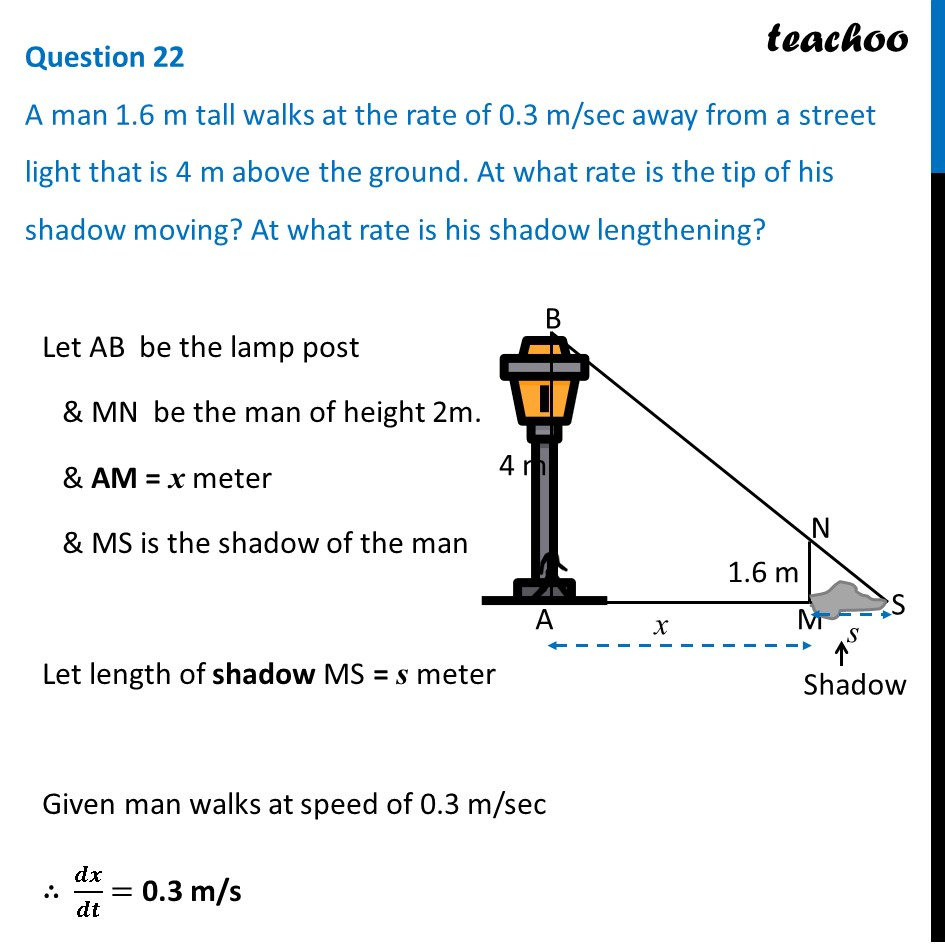googletag.cmd.push(function() { googletag.display('div-gpt-ad-1669298377854-0'); });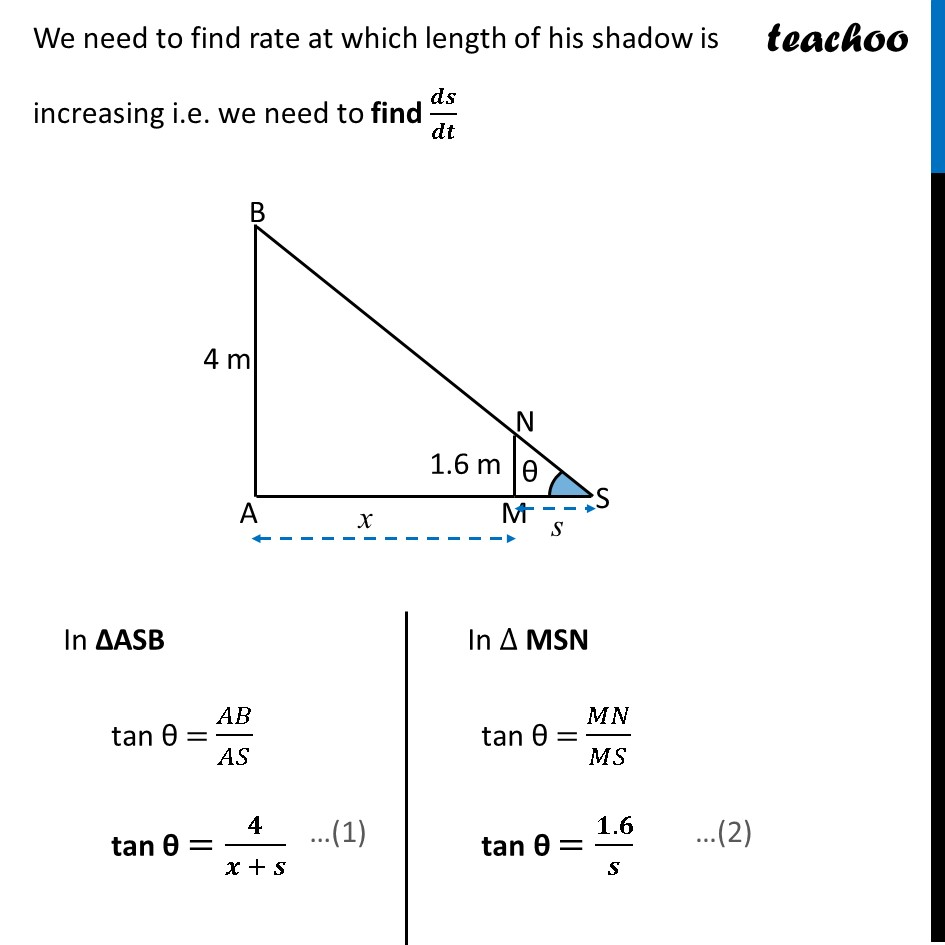(adsbygoogle = window.adsbygoogle || []).push({});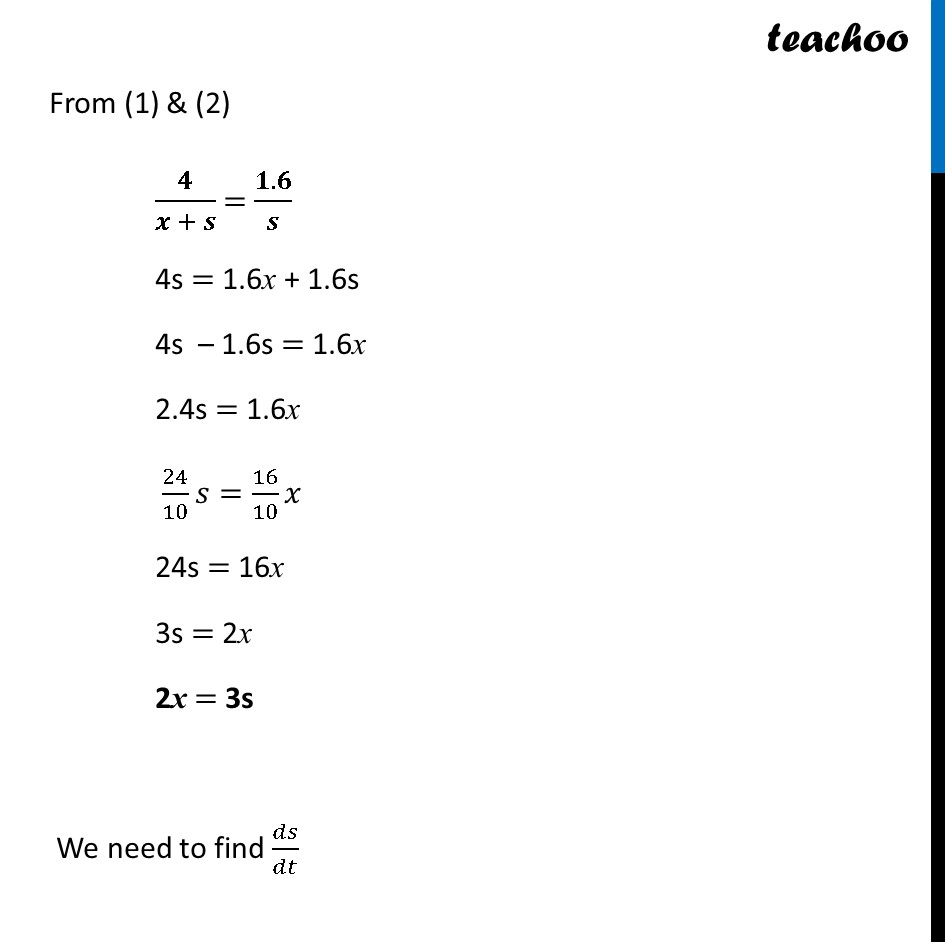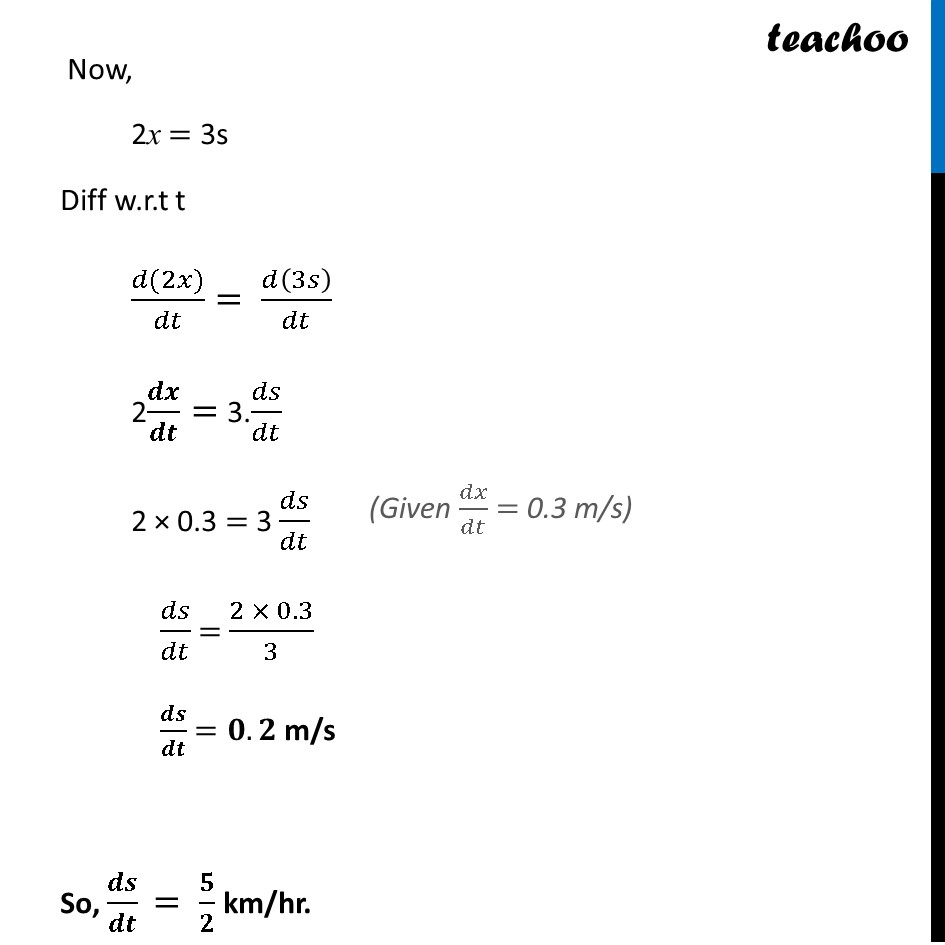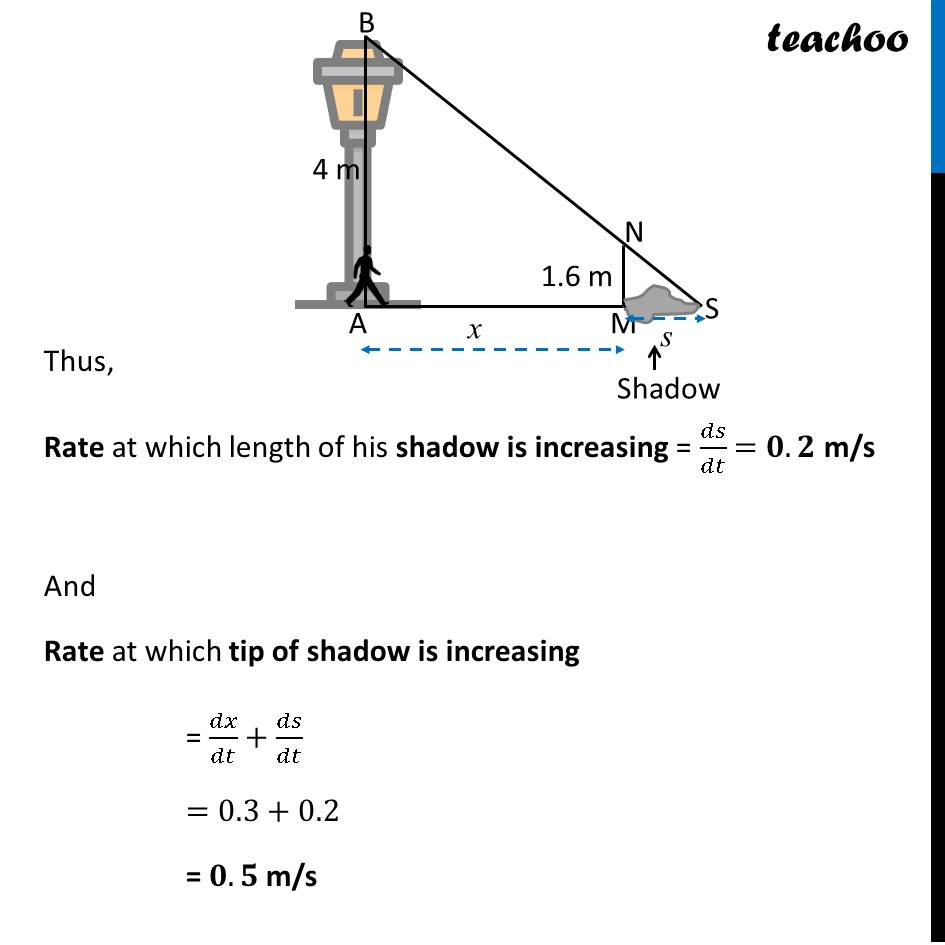Learn in your speed, with individual attention - Teachoo Maths 1-on-1 Class

### Transcript

Question 22 A man 1.6 m tall walks at the rate of 0.3 m/sec away from a street light that is 4 m above the ground. At what rate is the tip of his shadow moving? At what rate is his shadow lengthening? Let AB be the lamp post & MN be the man of height 2m. & AM = x meter & MS is the shadow of the man Let length of shadow MS = s meter Given man walks at speed of 0.3 m/sec ∴ 𝒅𝒙/𝒅𝒕 = 0.3 m/s We need to find rate at which length of his shadow is increasing i.e. we need to find 𝒅𝒔/𝒅𝒕 In ΔASB tan θ = 𝐴𝐵/𝐴𝑆 tan θ =𝟒/(𝒙 + 𝒔) In ∆ MSN tan θ = 𝑀𝑁/𝑀𝑆 tan θ =(𝟏.𝟔)/𝒔 From (1) & (2) 𝟒/(𝒙 + 𝒔) = (𝟏.𝟔)/𝒔 4s = 1.6x + 1.6s 4s – 1.6s = 1.6x 2.4s = 1.6x 24/10 𝑠=16/10 𝑥 24s = 16x 3s = 2x 2x = 3s We need to find 𝑑𝑠/𝑑𝑡 Now, 2x = 3s Diff w.r.t t (𝑑(2𝑥))/𝑑𝑡= 𝑑(3𝑠)/𝑑𝑡 2𝒅𝒙/𝒅𝒕= 3.𝑑𝑠/𝑑𝑡 2 × 0.3 = 3 𝑑𝑠/𝑑𝑡 𝑑𝑠/𝑑𝑡 = (2 × 0.3)/3 𝒅𝒔/𝒅𝒕 =𝟎.𝟐 m/s So, 𝒅𝒔/𝒅𝒕 = 𝟓/𝟐 km/hr.